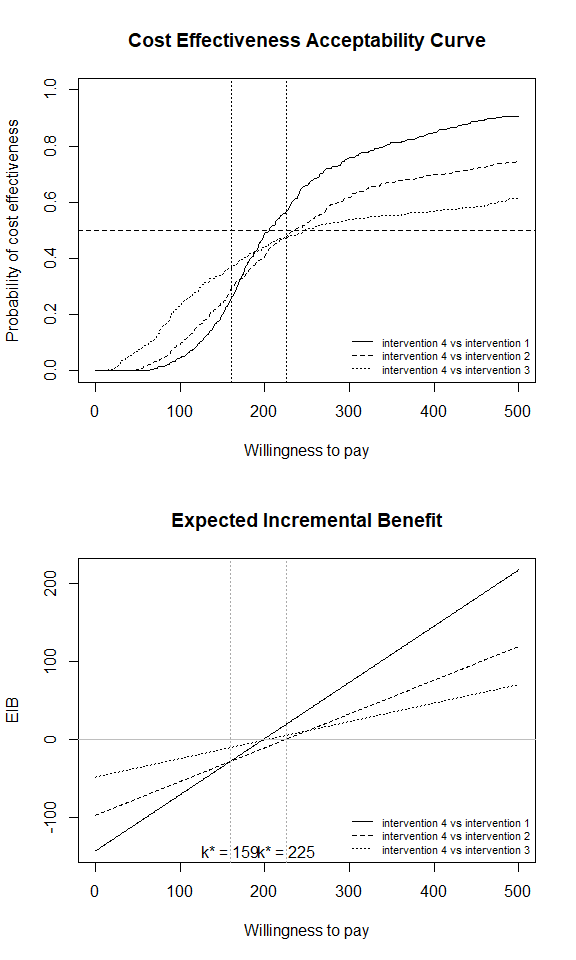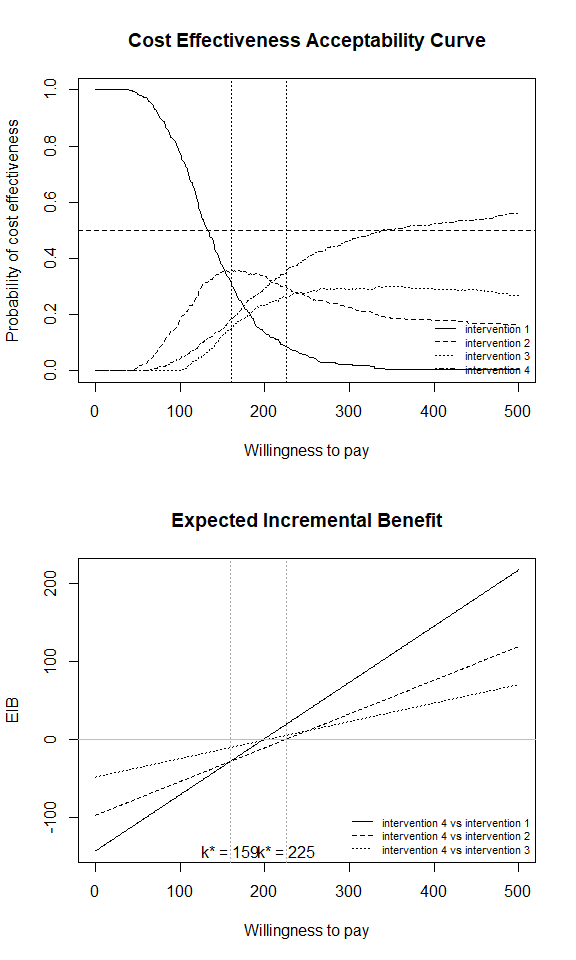# Paired vs Multiple Comparisons

## Introduction

The intention of this vignette is to show how to plot the CEAC and EIB plots depending on whether we consider all interventions simultaneously or pair-wise against a reference.

## Multiple interventions

This situation is when there are more than two interventions to consider. Incremental values can be obtained either always against a fixed reference intervention, such as status-quo, or for all comparisons simultaneously. We will call these a paired comparison or a multiple comparison.

### Against a fixed reference intervention

#### R code

This is the default plot for ceac.plot() so we simply follow the same steps as above with the new data set.

data("Smoking")
he <- bcea(eff, cost, ref = 4, Kmax = 500)
par(mfrow = c(2,1))
ceac.plot(he)
abline(h = 0.5, lty = 2)
abline(v = c(160, 225), lty = 3)
eib.plot(he, plot.cri = FALSE)### Pair-wise comparisons

#### R code

In BCEA we first we must determine all combinations of paired interventions using the multi.ce() function.

he.multi <- multi.ce(he)
par(mfrow = c(2, 1))
ceac.plot(he.multi)
abline(h = 0.5, lty = 2)
abline(v = c(160, 225), lty = 3)
eib.plot(he, plot.cri = FALSE)